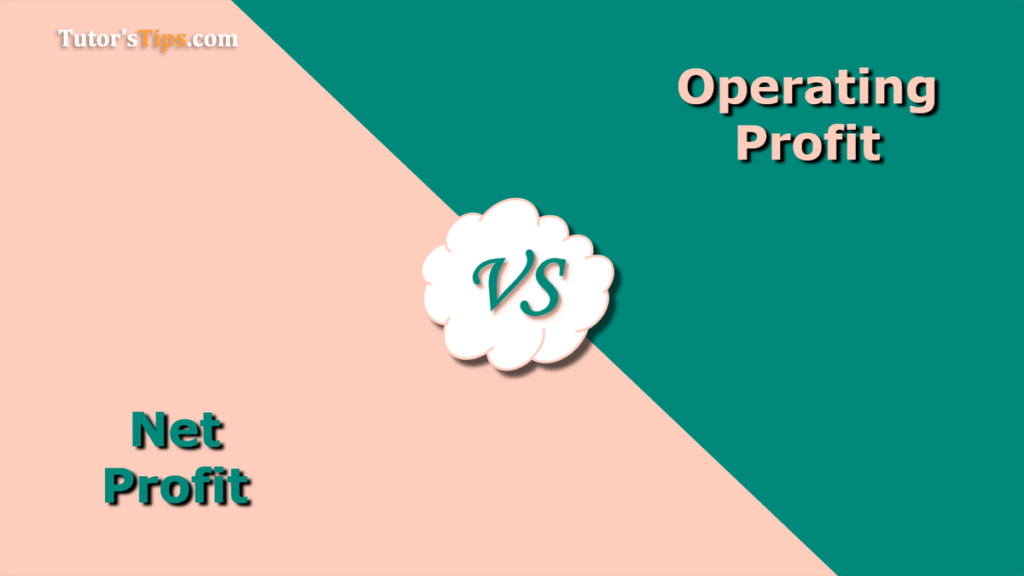# Difference between Operating Profit and Net ProfitTo know the Difference between Operating Profit and Net Profit first, we have to know the meaning and calculation method of both.

### Meaning of Operating Profit: –

Operating Profit is that part of the revenue which is left after deducting operating cost/expenses from the Gross Profit. Operating expenses/cost include all the cost incurred on all operative activities of the business. It is also known as Earning Before Interest and Taxation (EBIT).

#### 1. Base on Operating Revenue:

EBIT can be calculated from the total operating revenue with the following formula:

Operating Profit/EBIT = Operating Revenue – COGS – Operating Expense/cost – Depreciation – amortization”

#### 2. Base on Gross Profit: –

EBIT can be calculated from the Gross profit with the following formula:

“Operating Profit/EBIT = Gross  Profit – Operating Expense/cost – Depreciation – amortization”

#### Examples of Calculation of EBIT

##### Example 1 : –

As on 01/04/2019 A&b Co. ltd. have a stock of goods worth Rs. 35,000. The purchase goods worth Rs 1,50,000 and purchase return Rs 15,000 during the year and spent 2,000 on Carriage, 400 on loading. They sold goods for worth Rs. 5,35,000 and sale return Rs. 25,000 during the year and after that at the end of the year Rs 12,400 stock of goods left.

Other Expenses are salary 1,20,000/-, Electricity Rs. 50,000 and Depreciation of Plant and Machine Rs 10,000.

Calculate the EBIT of Mr X.

Solution: –

EBIT = Operating Revenue – COGS – Operating Expense/cost –
Depreciation – amortization”

First, calculate Cost of Goods Sold: –

COGS =  Opening Stock + Net Purchase + Direct Expenses – Closing Stock

35000 + (150000 – 25000) + 2000 + 400 – 12400

COGS = 1,50,000/-

Operating Expenses = Salary  + Electricity
=1,20,000 + 50,000
Operating Expenses =1,70,000/-

EBIT    = (5,35,000 – 25,000) – 1,50,000 – 1,80,000
= 5,10,000 – 2,30,000
EBIT = 2,80,000/-

### Meaning of Net Profit: –

Net Profit is that part of the revenue which is left after deducting total cost/expenses from the Net Sale or indirect cost/ expense from the Gross Profit. Indirect expenses/cost include all the cost incurred on the administrative expenses, selling & distribution, financing cost and Taxation.

“Net Profit = Gross Profit + Indirect Incomes – Indirect Expense/cost”

#### Example: –

Continue with the Last example, Further Mr X paid a salary to his employees 2,500/-, shop rent 1,000 and Shop lighting 200. Now calculate the Net Profit earned by Mr X.

Solution: –

Net Profit = Gross Profit – Indirect Cost/ Expenses

We already got Gross Profit = 18,500/-

SO,  18500 – 2500 -1000 – 200

Net Profit = 14,800/-

### Chart of Difference between Operating Profit and Net Profit: –

 Basis Operating Profit (EBIT) Net Profit(N.P.) Meaning Operating Profit is that part of the revenue which is left after deducting operating cost/expenses from the Gross Profit. NP is that part of the revenue which is left after deducting total cost/expenses from the Net Sale or indirect cost/ expense from the GP. Timing EBIT is calculated after GP NP is calculated after EBIT Purpose It is calculated to know the Actual profit earned from the sale of core products during the particular accounting period. It is calculated to know the actual profit earned from all activities during the particular accounting period. Stage It is calculated on the second stage of the final account. It is calculated on the third stage of the final account. Dependency Operating Profit is not dependent on EBIT NP is dependent on EBIT Advantage Helpful in control over the excess operating costs. Helpful in knowing the performance of the company in a financial year. Part EBIT is not a part of NP. NP is a part of EBIT.

### The conclusion of the Difference: –

Is simple we can say that, these are the important terms of accounting to know the actual grow or decline of an entity in the year by year comparison of these terms.